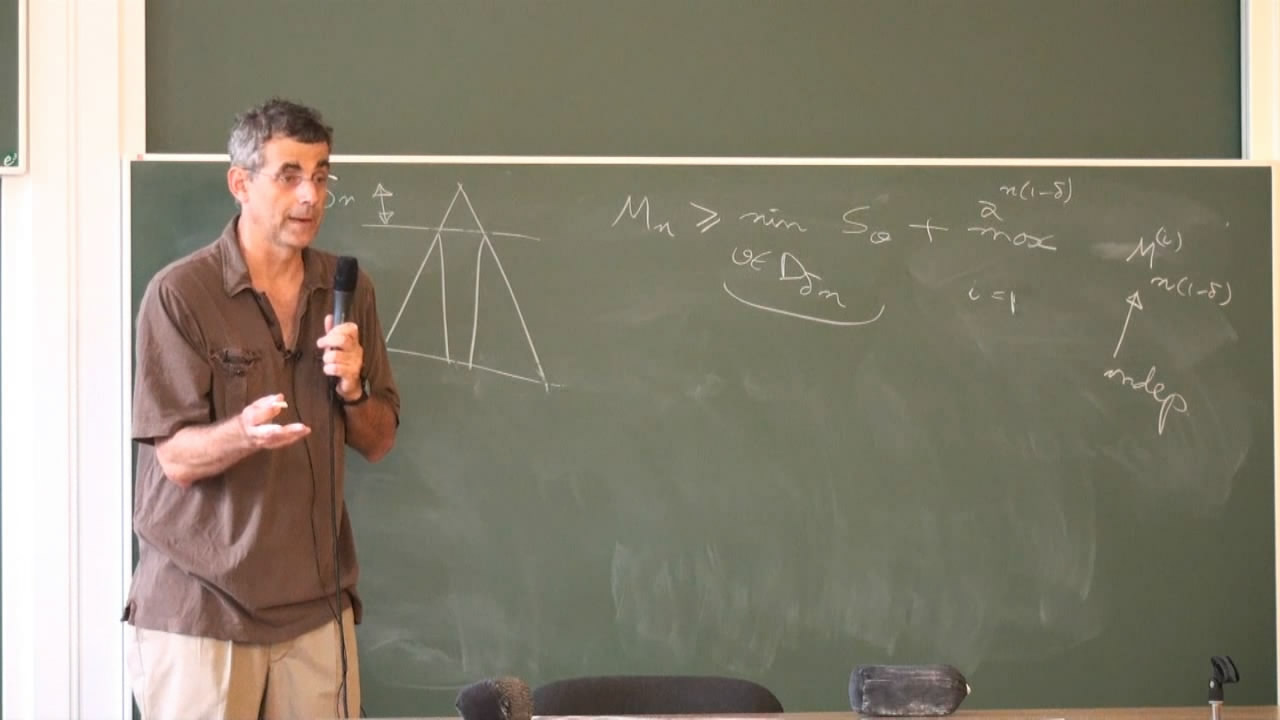# Branching random walks and maxima of Gaussian free fields

Курс Хит
Предмет:
Лектор:The model of branching random walks, and its close relative branching Brownian motion, describes the evolution of particles that undergo both random motion (diffusion) and branching. The analysis employs a mixture of analytical tools (the KPP equation, recursions, monotonicity) as well as probabilistic ones (changes of measures, first and second moment methods, large deviations), especially in dimension 1, to which this mini-course will be devoted. The classical main results are due to Fisher and Kolmogorov-Petrovsky-Piscounov, as well as McKean and Bramson.

There has been much recent progress concerning fine properties of the leading particles, as well as in exploiting the link with other problems involving random walk, and in particular cover time. Of particular interest is the link with a class of Gaussian fields called the (discrete) Gaussian free field, in dimension 2.

These lectures will present an introduction to both Branching random walks and Gaussian free fields. A rough plan is the following:

1. Definition of branching random walks, law of large numbers, recursions, tightness of maxima via the Dekking and Host argument, first and second moments methods, location of maximum. Introduction to the genealogy of the front. Inhomogeneous media and phase transitions.
2. Rough tree structures and cover times.
3. Gaussian free fields on graphs: definitions, fluctuations of maxima, basic inequalities (Borell-Tsirelson, Sudakov-Fernique). Subsequences and tightness: the Dekking-Host argument revisited. An introduction to the Dynkin isomorphism theorem.
4. Tightness of maximum for the 2D Gaussian free field: link with modified branching random walks, fluctuations.
5. Discussion of open problems.

Курс прочитан в рамках St. Petersburg School in Probability and Statistical Physics.

7